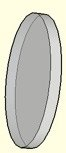## Thursday, September 28, 2006

### Next Lesson: Functions and RelationsToday's lesson was simply a chance for the students to begin working with their graphing calculators. I have a bunch of squirrely boys in the class, and they take to the TI-83+ like it was a Game Boy. It's amazing - I can show one of them how to do something once, and 5 minutes later half the class will be experts in it. I can give the same students directions about organizing their binders five times, verbally and in print, and there will still be tons of questions. It just goes to show how important motivation is in the learning process. One of my students keeps asking me where to find the games - after all, that's the whole point of getting one of these things!

Tomorrow, we begin the second unit, which focuses on functions. It's not going to be the most exciting lesson, as it will be primarily direct instruction.

The class starts with an introduction to functions, with the metaphor of a lens. I have two vertical number lines on a handout, with a picture of a lens (which I made in Google Sketchup) in the middle. The directions ask them to draw a stick figure on the left (the original image), with the feet at 0, the knees at 1, the hips at 2, the shoulders at 3, and the top of the head at 4. The lens is a "y = x^2" function, and they must map the projection onto the second number line by drawing guiding arrows from the inputs to the matching outputs. Later, when we do composition, I will use a double lens model to show how the projected image can then be used as the input of the second lens.

After that, we'll look at the definition of relations and functions, and I'll present them with functions in various forms: tables, arrow mappings, equations, graphs, and sets of ordered pairs. It's all about multirepresentational fluency, baby! We'll end that section with the vertical line test, and then learn about function notation.

I realized I screwed up on the homework I assigned from today's calculator lesson - I asked them to sketch a graph of f(x) = cos x. In my tutorial, I saw kids typing into their TIs: Y1 = F(x)cos(x). I forgot that I hadn't taught them yet what f(x) means. I'll just say what the freshmen say when they make a mistake: "just kidding!"